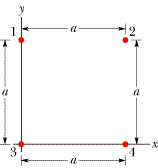Four Charged Particles in a Square

Homework Statement

In the figure below, four particles form a square. The charges are q1 = +Q, q2 = q3 = q, and q4 = -5.25Q. What is q/Q if the net electrostatic force on particle 1 is zero?F=k|q1||q2|/r2
a2+b2=c2

The Attempt at a Solution

I first drew a free body diagram of particle one with the forces of particles 2 and 3. Their resultant force needs to balance exactly the force of particle 4 in order for the net force on particle 1 to be zero.

Forces 2 and 3 both equal kQq/a2 so their resultant is 2kQq/a2.

The distance between particle 1 and 4 i called c. a2+b2=c2. In this case a and b are both a(according to the diagram) so: a2+a2=c2. Thus c=a$$\sqrt{2}$$.

I used this to get the force of 4 on 1: kQ|-5.25Q|/a$$\sqrt{2}$$2, which when simplified becomes 5.25q2k/2a2.

In setting this equal to the resultant of forces 2 and 3, I end up with 5.25/4=1.31=q/Q. This is not coming up as the right answer.

F2+F3=$$\sqrt{2}$$kQq/a2